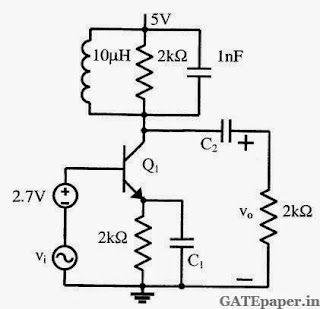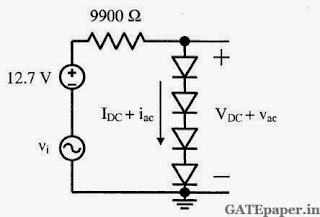### GATE 2011 ECE Video Solutions on Analog Circuits (Analog Electronics)

1. In the circuit shown below, capacitors C1 and C2 are very large and are shorts at the input frequency . vi is a small signal input. The gain magnitude |V0/Vi| at 10 M rad/sec isa. Maximum
b. Minimum
c. Unity
d. Zero

2. The circuit below implements a filter between the input current ii and the output voltage vo. Assume that the op-amp is ideal. The filter implemented is aa. Low pass filter
b. Band pass filter
c. Band stop filter
d. High pass filter

In the circuit shown below, assume that the voltage drop across a forward biased diode is 0.7 votls. The thermal voltage VT = KT/q = 25 mV. The small signal input vi = 100 cos(ωt) mV.3. The bias current IDC through the diodes is
a. 1 mA
b. 1.28 mA
c. 1.5 mA
d. 2 mA

4. The ac output voltage VAC is
a. 0.25 cos(ωt) mV
b. 1 cos(ωt) mV
c. 2 cos(ωt) mV
d. 22 cos(ωt) mV